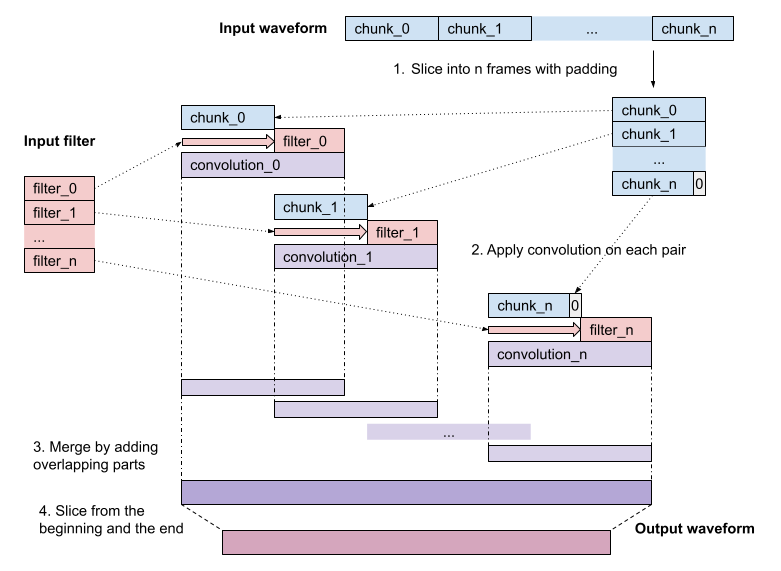Shortcuts

# torchaudio.prototype.functional.filter_waveform¶

torchaudio.prototype.functional.filter_waveform(waveform: Tensor, kernels: Tensor, delay_compensation: int = -1)[source]

Applies filters along time axis of the given waveform.

This function applies the given filters along time axis in the following manner:

1. Split the given waveform into chunks. The number of chunks is equal to the number of given filters.

2. Filter each chunk with corresponding filter.

3. Place the filtered chunks at the original indices while adding up the overlapping parts.

4. Crop the resulting waveform so that delay introduced by the filter is removed and its length matches that of the input waveform.

The following figure illustrates this.Note

If the number of filters is one, then the operation becomes stationary. i.e. the same filtering is applied across the time axis.

Parameters:
• waveform (Tensor) – Shape (…, time).

• kernels (Tensor) –

Impulse responses. Valid inputs are 2D tensor with shape (num_filters, filter_length) or (N+1)-D tensor with shape (…, num_filters, filter_length), where N is the dimension of waveform.

In case of 2D input, the same set of filters is used across channels and batches. Otherwise, different sets of filters are applied. In this case, the shape of the first N-1 dimensions of filters must match (or be broadcastable to) that of waveform.

• delay_compensation (int) – Control how the waveform is cropped after full convolution. If the value is zero or positive, it is interpreted as the length of crop at the beginning of the waveform. The value cannot be larger than the size of filter kernel. Otherwise the initial crop is filter_size // 2. When cropping happens, the waveform is also cropped from the end so that the length of the resulting waveform matches the input waveform.

Returns:

(…, time).

Return type:

Tensor

Tutorials using filter_waveform:Subtractive synthesis

Subtractive synthesis

## Docs

Access comprehensive developer documentation for PyTorch

View Docs

## Tutorials

Get in-depth tutorials for beginners and advanced developers

View Tutorials